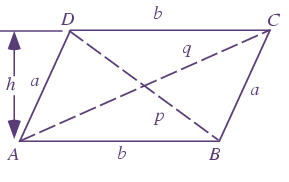# Diagonal of Parallelogram Formula

A parallelogram is a quadrilateral whose opposite sides are parallel and equal. The opposite sides being parallel and equal, forms equal angles on the opposite sides. Diagonals of a parallelogram are the segments which connect the opposite corners of the figure.Where,
p,q are the diagonals

a,b are the parallel sides

$\LARGE p=\sqrt{a^{2}+b^{2}-2ab\cos (A)}=\sqrt{a^{2}+b^{2}+2ab\cos (B)}$

$\LARGE q=\sqrt{a^{2}+b^{2}+2ab\cos (A)}=\sqrt{a^{2}+b^{2}-2ab\cos (B)}$

$\LARGE p^{2}+q^{2}=2(a^{2}+b^{2})$

### Solved Examples

Question 1:

Find the diagonal of a parallelogram with sides 3 cm, 5 cm and angle 45 degrees ?

Solution:

Given a = 3 cm
b = 5 cm
angle A = 45°
Formula of diagonal is,

q =

$$\begin{array}{l}\sqrt{a^{2}+b^2-2ab cosA}\end{array}$$

q =

$$\begin{array}{l}\sqrt{3^{2} + 5^2 – 2\times 3 \times 5 cos 45}\end{array}$$
q =
$$\begin{array}{l}\sqrt{34 – 30\times 0.707 }\end{array}$$

q = √12.79

=3.576 cm

Diagonal  of parallelogram = 3.576 cm.